# Outdoor Localization for a Mobile Robot under Different Weather Conditions Using a Deep Learning Algorithm

Outdoor Localization for a Mobile Robot under Different Weather Conditions Using a Deep Learning Algorithm
Control and Systems Engineering Department, University of Technology-Iraq, Baghdad 10066, Iraq

Corresponding Author Email:
Page:
1-9
|
DOI:
https://doi.org/10.18280/jesa.560101
7 November 2022
|
Accepted:
15 January 2023
|
Published:
28 February 2023
| Citation

OPEN ACCESS

Abstract:

A fundamental issue in robotics is the precise localization of mobile robots in uncertain environments. Due to changing environmental patterns and lighting, localization under difficult perceptual conditions remains problematic. This paper presents an approach for locating an outdoor mobile robot using deep learning algorithms merge with 3D Light Detection and Ranging LiDAR data and RGB-D image. This approach is divided into three levels. The first is the training level, which involves scanning the localization area with a 3D LiDAR sensor and then converting the data into a 2.5D image based on the Principal Component Analysis. The testing is the second level in the Intensity Hue Saturation process. Then, the RGB and Depth images are combined to create a 2.5D fusion image. These datasets are trained and tested using Convolution Neural Networks. The K-Nearest Neighbor algorithm is used in the third level is the classification. The results show that the proposed approach is better in terms of accuracy of 97.46% and the Mean error distance is 0.6 meters.

Keywords:

CNN, deep learning, K-Nearest neighbors algorithm, principal component analysis, mobile robot localization

1. Introduction

Mobile robots are equipped to perform human service roles, either because the tasks are challenging or hazardous  or simply because of ease of use . Mobile robots are used in agriculture , military applications , and medical activities. For example, the outbreak of the coronavirus illness (COVID-19) in 2020 accelerated the process to industries the autonomous robots . One of the most important aspects of mobile robots is navigation . In some conditions, localization provided success in navigation.

The problem of localization in the mobile robot is the estimation in the operating area of the location and orientation of the autonomous mobile robot. The location of the robot depends upon the sensor used. Odometry, RGB, Light Detection and Ranging (LiDAR), Global Position System (GPS), laser radar, ultrasonic, infrared, and microwave are the sensors widely used for mobile robots .

Due to varying environmental conditions, some methods declined to classify a mobile robot's position accurately. Furthermore, specific sensors, for example RGB, did not perform admirably in an outdoor setting where light is empathetic. Other approaches are affected in rainy or textured environments. As a result, a system dependent on 3-D data and a DL technique was proposed to obtain precision and durability in identifying the precise position of the mobile robot in various weather conditions. In addition, a robust CNN architecture with thousands of datasets has been proposed with differences in the data collection environment.

There are three levels in the suggested process. Each level contains a variety of activities, including training level, testing level, and classification level. The PCA technique is used to convert a 3D LiDAR point cloud scan into a 2.5-D image during the training level. The Convolution Neural Network (CNN) algorithm is used to extract features from the 2.5-D images., Then, to include them in the classification level, all features and point cloud data are saved as a matrix. This is for determining the proper ground location of a mobile robot. Image fusion is performed at the testing level by merging two pictures, RGB and Depth, into a single RGB-D fusion image and then features extraction from the RGB-D fusion image using CNN. In the classification level, the tested image is classified using the K-Nearest Neighbors algorithm to identify the location of a mobile robot.

The novelty of this paper is to improve outdoor localization in mobile robots using 3-D data from LiDAR and RGB-D, using the proposed system of PCA method, KNN algorthim, and deep learning algorithm.

This paper is structured as follows: The related work in Section 2. In section 3, the material and methodology are presented. the proposed method is outlined in details in section 4. The experimental results and discussion are presented in Section 5. Finally, section 6 gives the conclusion.

2. Related Work

Autonomous navigation is one of the most difficult tasks a mobile robot can perform. To navigate successfully, a mobile robot must be skilled in four critical elements of autonomous navigation: perception, localization, recognition, and motion control. As a result, significant progress has been made. This section will provide a summary of the most commonly used outdoor localization techniques for mobile robots. It will also discuss the various sensor vision techniques used to improve the overall precision and efficiency of the localization method, as well as the benefits and challenges associated with each.

In the outdoor environment, GPS is one of the usual localization solutions. However, these solutions are inaccurate or available in environments such as (tunnels, caves, tall buildings, and under trees) . It can lead to errors in a few meters, which is unacceptable to robot driving. Furthermore, a travelling robot must move in a complicated world of possible challenges, even though it does not always have previous knowledge of its surroundings .

As a result of these issues, vision is already the most often used sensor for the outdoor position [10, 11]. However, optimizing robotics performance through the application of Machine Learning (ML) technologies has created new difficulties in ML. There has been an increase in interest and dedication in developing ML methods for robotics systems that rely on computer vision in recent years .

Because illumination is strongly dependent on the terrain, such as (sunshine, rain, clouds), outdoor lighting changes are a major concern for visibility. RGB cameras alone may not be sufficient. Current sensors, such as portable LiDAR or RGB-D cameras, add depth information to RGB imagery, opening up new possibilities for developing robust and practical applications [13-15].

The research community focuses on Deep Learning (DL) in Localization of mobile robots. Two approaches for outdoor localization were presented by Nilwong et al. , with an increasing focus on deep learning and landmark recognition. The first access uses a Faster Regional-Convolutional Neural Network to identify landmarks in the recorded image (Faster R-CNN). Then, using the landmarks as input, a Feedforward Neural Network (FFNN) is used to estimate robot position coordinates and compass direction. Unfortunately, the failure rate of orienting was extremely high, and the outcomes were poor. The following factors contribute to high orientation errors: - The proposed localization methods only needed a small amount of data; - During data collection, there were very few environmental variations. Debeunne and Vivet  presented a comprehensive study into visual-LiDAR Simultaneous Localization and Mapping SLAM. LiDAR-based SLAM provides exact 3D details about the environment. Still, it is time-intensive and relies on insecure scan-matching techniques, and in rainy or textured environments, LiDAR-SLAM performs poorly.

For example, several researchers have experimented with determining the robot's position in various weather environments. Rawashdeh et al.  used infrared, visual odometry, and depth cameras. This approach was weak, and it could not be used independently. Outdoor navigation localization using simply a stereo camera was proposed by Irie et al. , but the findings revealed some mistakes in the meters. Moreover, it failed under the worst circumstances. Owing to the camera's small dynamic range, direct illumination, for example, will blacken or whiten a significant chunk of the acquired image. This scenario cannot see good edge points, resulting in considerable motion measurement errors.

3. Methodology

To train the deep learning network and obtain the 3D dataset, two sensors are used mounted with the mobile robot; LiDAR and RGB with Depth (RGB-D) sensor.

3.1 Point cloud LiDAR sensor

The data obtained by a LiDAR is used to create 3D models and maps of structures and environments. LiDAR determines the structure by measuring the time it takes for signals to bounce off surfaces and return to the scanner . After analysing and organising the individual readings, the LiDAR data becomes point cloud data. The point clouds are vast arrays of 3D elevation points with x, y, and z coordinates . A standard 3D LiDAR is from the other hand, may acquire surroundings data with a vertical Field of View (FOV) of 30 (±15) º and a horizontal FOV of 360º at a scanning rate of about 10 Hz . High resolution allows the LiDAR to gather a large amount of valuable data in a region of long ranges. LiDAR is commonly used in robot systems because of these advantages .

3.2 Reduction 3D LiDAR point cloud to 2.5D image

One of the most well-known and widely utilized methods is Principal Component Analysis (PCA). Its premise is straightforward: limit the dimensionality of a dataset while retaining as much 'variability' (i.e., statistical information) as possible . Solving the covariance matrix obtains the major components. The PCs of the PCA space are calculated in this method in two steps. The data matrix's covariance matrix (X) is constructed first. Second, the covariance matrix's eigenvalues and eigenvectors are determined. The covariance matrix is asymmetric and always positive semi-definite matrix (i.e., X= XT). The variable variance is represented by the diagonal values xi, I= 1,..., M of the covariance matrix, whereas the off-diagonal entries as illustrated in Equation (1), represent the covariance between two separate variables. A positive covariance matrix value shows A positive correlation between two variables is indicated by a positive value, a negative correlation is indicated by a negative value, and a zero value indicates that the two factors are uncorrelated or statistically independent .

$\left(\begin{array}{cccc}\operatorname{Var}\left(\mathrm{x}_1, \mathrm{x}_1\right) & \operatorname{Cov}\left(\mathrm{x}_1, \mathrm{x}_2\right) & \ldots & \operatorname{Cov}\left(\mathrm{x}_1, \mathrm{x}_{\mathrm{M}}\right) \\ \operatorname{Cov}\left(\mathrm{x}_2, \mathrm{x}_1\right) & \operatorname{Var}\left(\mathrm{x}_2, \mathrm{x}_2\right) & \ldots & \operatorname{Cov}\left(\mathrm{x}_2, \mathrm{x}_{\mathrm{M}}\right) \\ \vdots & \vdots & \ddots & \vdots \\ \operatorname{Cov}\left(\mathrm{x}_{\mathrm{M}}, \mathrm{x}_1\right) & \operatorname{Cov}\left(\mathrm{x}_{\mathrm{M}}, \mathrm{x}_2\right) & & \operatorname{Var}\left(\mathrm{x}_{\mathrm{M}}, \mathrm{x}_{\mathrm{M}}\right)\end{array}\right)$                (1)

The eigenvalues ($\lambda$) and eigenvectors (V) of the covariance matrix are calculated based :

$V \Sigma=\lambda V$             (2)

The first principal component has the most giant variance and is represented by the eigenvector with the highest eigenvalue. Each eigenvector corresponds to a single primary component. The eigenvectors depict the directions in the PCA space .

3.3 Ground removal by rotation around Z-axis

In 3D, there are a variety of techniques to express rotations. Euler angles, quaternions, and rotation matrices are common representations. Even though they require various parameters, these representations can describe rotations with three degrees of freedom [22, 23]:

$\mathrm{R}_{\mathrm{z}}=\left[\begin{array}{ccc}\cos (\theta) & -\sin (\theta) & 0 \\ \sin (\theta) & \cos (\theta) & 0 \\ 0 & 0 & 1\end{array}\right]$           (3)

$\mathrm{R}_{\mathrm{x}}=\left[\begin{array}{ccc}1 & 0 & 0 \\ 0 & \cos (\theta) & -\sin (\theta) \\ 0 & \sin (\theta) & \cos (\theta)\end{array}\right]$            (4)

$\mathrm{R}_{\mathrm{y}}=\left[\begin{array}{ccc}\cos (\theta) & 0 & \sin (\theta) \\ 0 & 1 & 0 \\ -\sin (\theta) & 0 & \cos (\theta)\end{array}\right]$             (5)

3.4 RGB-D camera

Because of popular RGB-D sensors such as Microsoft's Kinect, showing 3D data as RGB-D images has really become popular in recent years. RGB-D data provides a 2.5D representation of the captured 3D object by including a depth map (D) as well as 2D color information (RGB). Although cheap, RGB-D data are simple yet effective representations of 3D objects that can be used for various tasks such as identity recognition, pose regression, and scene reconstruction [24, 25].

3.5 Fusion image by (IHS) Transformations

Image fusion is a technique used to extract useful data from many input photographs and merge it to create a new output image that is more descriptive and useful than the sum of the input images . Image fusion reduces data size, keeps vital features, and provides a more accessible image .

The IHS methodology has become a standard process in image processing for color enhancement, feature enhancement, pixel size optimization, and the merging of various data sets. The goal of combining high-resolution and hyperspectral remotely sensed photos is to ensure spectral data while also including high - spatial detail information, making the fusion significantly more suitable for IHS treatment . Because the RGB to IHS conversion model employs a 3x3 matrix as its transform kernel, most literature considers IHS to be a third-order process. Most literature considers IHS to be a third-order process. Many published studies indicated that different IHS transformations are used, which have significant variations in the matrix values, as described below. R = Red, G = Green, and B = Blue were used in this analysis. I = Intensity, H = Hue, S = Saturation, and V1, V2 = Cartesian hue and saturation elements .

$\begin{gathered}{\left[\begin{array}{c}I \\ V_1 \\ V_2\end{array}\right]=\left[\begin{array}{ccc}\frac{1}{\sqrt{3}} & \frac{1}{\sqrt{3}} & \frac{1}{\sqrt{3}} \\ \frac{-1}{\sqrt{6}} & \frac{-1}{\sqrt{6}} & \frac{2}{\sqrt{6}} \\ \frac{-1}{\sqrt{2}} & \frac{1}{\sqrt{2}} & 0\end{array}\right]\left[\begin{array}{l}R \\ G \\ B\end{array}\right] H=\tan ^{-1}\left(\frac{\mathrm{V} 2}{\mathrm{~V} 1}\right) \mathrm{S}=} \sqrt{V_1^2+V_2^2}\end{gathered}$            (6)

The RGB and depth pictures are merged in the precise location to form the 2.5D image in IHS methods. The color-carrying data is detached from the intensity variable in a color picture (Hue and Saturation). The IHS color is created by utilizing geometrical formulas to convert RGB points into matching points .

3.6 Deep learning algorithms

Machine learning has taken a powerful turn in recent years, thanks to the rise of Artificial Neural Networks . One of most impressive forms of ANN architecture is CNN . The CNN is a hybrid of artificial neural networks and cutting-edge deep learning techniques. CNN's are at the heart of spectacular developments in deep learning. For decades, this artificial neural network has been used to perform various image detection tasks. CNN has recently piqued the interest of researchers from around the world, as it has demonstrated impressive results in a variety of computer vision and machine learning tasks . As depicted in Figure 1, CNNs are built up of three layers: Convolution, pooling, and fully connected layers. Convolution and pooling are the first two layers that extract features, whereas the third, a fully connected layer, maps specific features into the outcome, such as classification. A convolution layer, a type of linear operation, is a crucial aspect of CNN. It is made up of a set of mathematical operations such as convolution. Image pixels are stored in a two-dimensional matrix .

## 1.png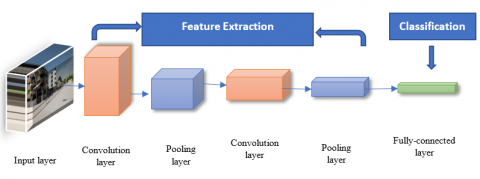Figure 1. The architecture of CNN 

3.7 K-Nearest Neighbour (K-NN) algorithm

For data classification tasks, distance-based algorithms are commonly used. One of the most widely used distance-based algorithms is the K-Nearest Neighbor classification (K-NN). This classification calculates the distances between the testing machine and the training data to decide the final classification performance. With numerical results, the standard K-NN classifier works well . A popular distance-based classification method is the K-Nearest Neighbour's (K-NN) classification technique. The standard K-NN classification algorithm recognises the K-Nearest Neighbor(s) and categorizes numerical data records by measuring the distance between both the test sample and all training samples using the Euclidian distance .

1. Calculate the number of closest neighbours (K values); see Figure 2.

2. As indicatedin Figure 3, find the distance between both the testing data and one of the training examples.

The proximity between $\mathrm{A}$ and $\mathrm{B}$ in Euclidean$\text { distance }=\sqrt{\left(X_2-X_1\right)^2+\left(Y_2-Y_1\right)^2}$         (7)

## 2.png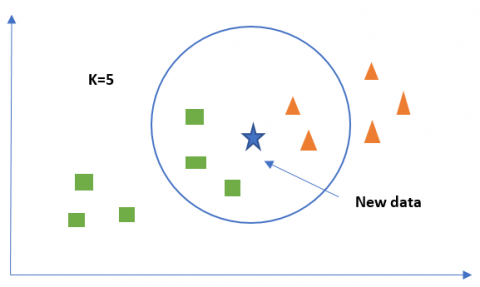Figure 2. K-Nearest neighbor principle 

3. Sort the distance using the K-th minimal distance to find the nearest neighbours.

4. Make a list of the categories of your nearest neighbours.

5. Use the simple majority of the group of closest neighbors as the additional data object's prediction value. neighbours.

## 3.pngFigure 3. The Euclidian distance to measure the distance between two data 

3.8 Measuring the error

To calculate the error of an approximate arithmetic circuit. The Error Rate (ER), Mean Square Error (MSE), and Mean Error Distance (MED) are used to calculate the error rate. For example, the discrepancy between the estimated and real sums S* and S is known as the error of distance (ED) :

$E D=\left|S^*-S\right|$          (8)

The error rate is the number of input configurations for which the predicted adder produces wrong outputs (ER). As a result, a non-zero error gap can be calculated as:

$\mathrm{ER}=\mathrm{P}(\mathrm{ED} \neq 0)$            (9)

The (MED) is the average of all error distances. The Mean Squares of Error (MSE) overall error distances is calculated as :

$\mathrm{MED}=\mathrm{E}[\mathrm{ED}]=\sum_{\mathrm{ED}_{\mathrm{i}} \in \Omega} \mathrm{ED}_{\mathrm{i}} \mathrm{P}\left(\mathrm{ED}_{\mathrm{i}}\right)$            (10)

$M S E=E\left[E D^2\right]=\sum_{\mathrm{ED}_i \in \Omega} E D_{\mathrm{i}}^2 \mathrm{P}\left(\mathrm{ED}_{\mathrm{i}}\right)$            (11)

where, Ω is the total number of error distances.

MSE is calculated as follows: if n predictions are made from a sample of n data, Y is the variable being forecasted, and Y ̂ is the predicted value :

MSE $=\frac{1}{n} \sum_{i=1}^n\left(Y_i-\widehat{Y}_i\right)^2$                 (12)

4. Proposed Outdoor Localization System

To improve the efficiency and reliability of a localization system, the proposed method uses 3-D data to determine the position of a mobile robot under various weather conditions. The proposed approach is depicted in Figure 4, which is formed by two sensors LiDAR and RGB-D position on a mobile robot for data collection for training and testing. This paper's proposed method is divided into three levels. There are several operations at the training level, testing level, and classification level. During training, the LiDAR sensor performs a scan to collect 3-D dataset. The PCA method converts a 3-D point cloud to a 2.5-D image. Then the feature is extracted from 2.5-D images using the CNN algorithm. A matrix is used to store all featured data, pre-processing and point cloud data. The RGB and Depth sensors are used in the testing level to obtain two images of the exact location. Using the IHS method, merge RGB images with Depth into an RGB-D image to create a 2.5-D fusion image. The CNN algorithm extracts the features from a 2.5-D RGB-D image. Following that, all of the feature data is arranged in a matrix. To determine the correct position of the mobile robot, The test data is categorized alongside the training data in the classification level of the K-NN classifier.

## 4.png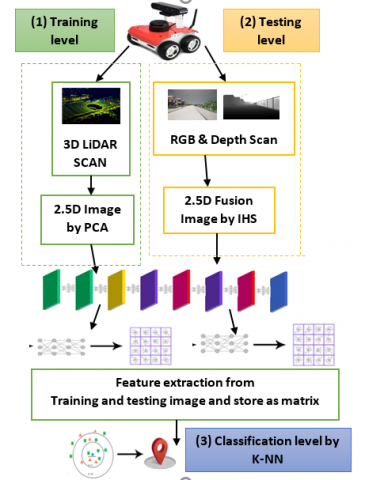Figure 4. Proposed outdoor localization system

5. Experimental Results

5.1 Collected datasets

Using supervised learning, typically, a large amount of data is required to train a neural network. To train and test the proposed system, a dataset that included Image data in different weather conditions, depth images, and LiDAR data with corresponding mode labels was used. Seeing as this was not realized in the public domain, it was decided to employ a simulator to generate the data required. Simulated results are created using the CARLA driving simulator. CARLA is a testing simulator for autonomous vehicles (open-source simulator) . CARLA was explicitly built to support the production, training, and testing of self-driving automated systems. CARLA also offers available digital assets (urban layouts, homes, and automobiles) built specifically for this purpose and can be widely accessed [38, 39]. A camera sensor can be attached to a mobile robot in CARLA to capture images at a fixed frame rate. As seen in Figure 5, the camera sensor can produce images in both RGB and Depth.

## 5-1.png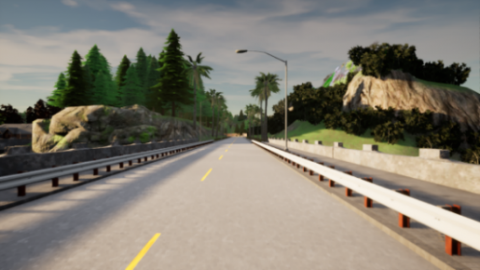(a) RGB image

## 5-2.png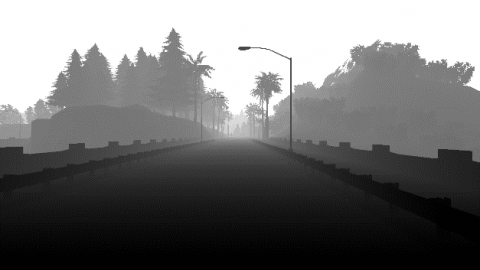(b) Depth image

Figure 5. The RGB image corresponds to the Depth map (photos from Carla open-source simulator)

The simulator is capable of simulating a variety of weather conditions. Rain with puddles light reflected, cloud cover that darkens the ambience, and simulated sunny weather that produces fake lens flares whereas the sun is in the frame are all examples. In addition, data on a variety of environmental conditions is collected , seen in Figure 6.

## 66.png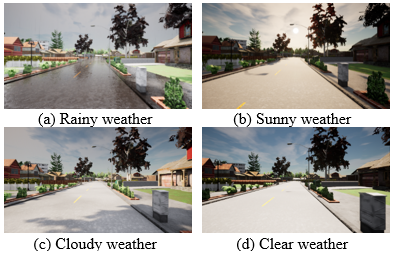Figure 6. Image visualization in a variety of weather conditions (photos from Carla open-source simulator)

LiDAR sensors can be reproduced in CARLA. The upper and lower farms of view, as well as the number of channels, total size, and the number of points in each direction can be customized. During scan capture, the simulation region can be frozen, resulting in a 360-degree scan without the need for velocity adjustments. Figures 7 and 8 are two examples of this.

To create the data sets used in this study, a mobile robot equipped with LiDAR sensor and RGB-D camera was set to travel around a map on autopilot. The data set encompasses roughly 120,000 m2 of a map, including the suburban neighbourhoods, downtown area, and wooded areas. Two training data sets were obtained, one with 15000 images, 5000 images for each weather condition and Depth picture pairs, and 46,741 LiDAR frames. As seen in Figure 9, this city district is divided into nine streets with a street between them.

Each street has two sections: the begin and the end, as seen in Table 1.

## 7.pngFigure 7. LiDAR scan as a point cloud (photos from Carla open source simulator)

## 8.png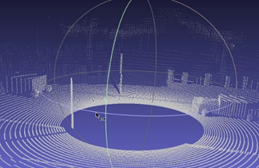Figure 8. LiDAR analysis after MeshLab display 

## 9.png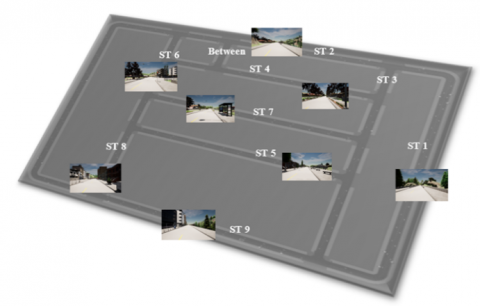Figure 9. Carla simulator's suggested street numbers

5.2 CNN localization

The CNN for localization has a total of 12 layers. With a brief training period, a 12-layer CNN with a 224 x 224 input image was designed. The optimizer Stochastic Gradient Descent with Momentum (SGDM) was used in this network. Table 2 shows the analysis result of the network design. With the K-NN classifier, there are 16 classes based on the number of streets identified in Figure 9.

Figure 10 illustrates the configuration of the implemented CNN.

## 10.png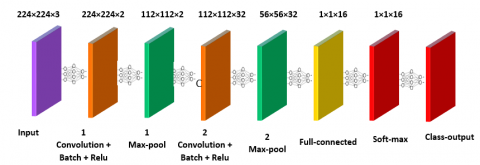Figure 10. CNN localization with 12-layers

Table 1. Division of streets and data for each street

 Item The name of the street No. of LiDAR frame estimates for per street Each street has a certain number of test images (each weather conditions) Cloudy Rainy Sunny 1 The begin of street 1 750 20 15 15 2 The end of street 1 1600 20 36 35 3 The begin of street 2 900 20 20 15 4 The end of street 2 3300 70 60 80 5 street 3 750 10 15 15 6 The begin of street 4 1750 36 30 36 7 The end of street 4 1900 30 20 28 8 The begin of street 5 2650 55 45 60 9 The end of street 5 1300 20 30 35 10 Street 6 950 30 15 15 11 The begin of street 7 3250 40 95 50 12 The end of street 7 1550 28 65 30 13 Street 8 1600 40 30 40 14 The begin of street 9 1200 15 30 15 15 The end of street 9 1300 10 45 15 16 Between street 1000 30 25 30

Table 2. Analysis result for the CNN architecture

 Name Type Activations Learnables 1 Imageinput 224×224×3 image with ‘zerocenter’ normalization Image input 224×224×3 - 2 CONV_1 2 3×3×2 convolutions with stride [1 1] and padding [1 1 1 1] Convolution 224×224×2 Weights 3×3×3×2 Bias       1×1×2 3 Batchnorm_1 Batch normalization with two channels Batch Normalization 224×224×2 offset   1×1×2 scale    1×1×2 4 Relu_1 ReLU ReLU 224×224×2 - 5 Maxpool_1 2×2 max pooling with stride [2 2] and padding [0 0 0 0] Max Pooling 112×112×2 - 6 CONV_2 32 3×3×2 convolutions with stride [1  1] and padding [1  1  1  1] Convolution 112×112×32 Weights 3×3×2×32 Bias      1×1×32 7 Batchnorm_2 Batch normalization with 32 channels Batch Normalization 112×112×32 offset   1×1×32 scale    1×1×32 8 Relu_2 ReLU ReLU 112×112×32 - 9 Maxpool_2 2×2 max pooling with stride [2 2] and padding [0 0 0 0] Max Pooling 56×56×32 - 10 FC 16 fully connected layer Fully Connected 1×1×16 Weights 16×100352 Bias       16×1 11 Softmax softmac Softmax 1×1×16 - 12 Classoutput Crossentropex with ‘between’ and 15 other classes Classification output - -

## 11.jpg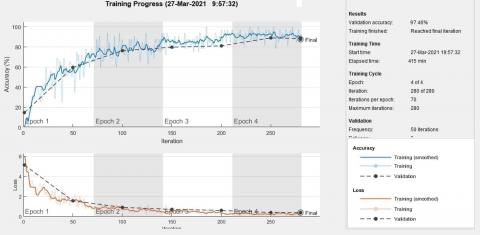Figure 11. The result of training time expended and training accuracy

5.3 Network training and analysis

The MatLab code was created on a PC equipped with a Core (TM) Intel (R) i5-8250U processor. 1.80 GHz RAM 8 GB, UHD Graphics 620. It took four epochs to process the dataset containing 46,741 LiDAR frames. Each epoch has 70 iterations, with a maximum of 280 iteration numbers possible and a learning period rate of 3*10-4. The preparation took 415 minutes, containing 70% of the dataset for training and 30% of the test dataset. As seen in Figure 11, the accuracy obtained is 97.46%.

5.4 Result of IHS transformation

When merging RGB and Depth images, the IHS method has an impact. The Intensity of a pixel in a depth picture varies based on its brightness. Figure 12 shows how the Hue, Saturation, and Intensity combine in the 2.5-d images in various weather conditions. The change in the 2.5D images is noticed for these three different weather conditions, which led to the strength of the approach in identifying the mobile robot's correct position in these weather changes.

## 12.pngFigure 12. Creating a 2.5D image by merging two RGB with different conditions and depth images

5.5 Evaluation results

To check the performance of the proposed approach of PCA method with IHS and using the K-NN algorithm, it is necessary to obtain a high-quality and precise localization sample that can be compared to reality on the ground. Tested nine states with random locations in the city under different weather conditions are listed in Table 3:

Table 3. Summary of test results in various weather conditions (Cloudy, Rainy and Sunny weather)

 State The correct localization prediction MSE 1 Between streets 0.0432 2 Beginning of street 1 0.0472 3 Beginning of street 2 0.0421 4 Street 6 0.0360 5 End of street 9 0.036 6 Street 3 0.0216 7 End of street 1 0.0545 8 End of street 5 0.0678 9 Street 6 0.036 Mean error 0.0427

Table 4. A comparison of the proposed method to other methods

 Method Average error Maximum error Training time Data set Fingerprinting positioning method + IEEE802.11a platform, i.e., 5 GHz in ISM band  20.12 m 20.12 m -- -- Particle filter based on Monte Carlo Localization  0.6 m 2.7 m - 16530 images ICP+ PointNetLK+ GoogleNet  18.3 m 131.6 m 4200 minutes 10400 images Proposed method CNN +PCA +K-NN 0.6 m 1.09 m 415 minutes 46741 frame LiDAR

CARLA achieved a frame rate of 30 frames per second (FPS) in one meter to calculate the Mean Error Distance. From equations (10 and 11), MED = 0.6 meters.

The proposed approach is superior to the research method is depicted in Table 4.

Table 4 reference 40 used the technique positioning system WifiLOC, which is currently being developed as a research tool at the University of Zilina. WifiLOC estimates a mobile device's location using RSS information from nearby Wi-Fi APs. The method is built on the fingerprinting positioning algorithm and earlier RSS data. The proposed approach does not rely on a picture of deep learning technologies, so the error rate is respectively high. In reference 17, Only use a stereo camera for vision-based localization in outdoor environments. A particle swarm optimization based on Monte Carlo Localization is used in the localization process, which fails in extreme cases. For example, due to the camera's restricted dynamic range, direct sunlight will saturate a significant portion of the captured image to black or white. Researchers discovered that this problem is equivalent to wheel slip in wheel odometry and categorized it as an abducted robot problem to overcome this limitation. It also notes that the average error is almost equal to the proposed method, but the maximum error is 2.7 m, and the proposed method does not exceed 1.09 m. While in reference 23, the (ICP) algorithm was used, the PointNetLK network was utilized in registration, and GoogleNet was used for the RGB-D Neural network. Also, notice that average error and training time are very high compared to the proposed method. The proposed system used a deep learning technique that took 415 minutes to train. Although the dataset was 46,741 LiDAR frames to obtain the accuracy of 97.46%, MSE equals 0.0427, and the Mean Error of Distance equals 0.6 meters using the PCA method for the fusion picture IHS is used the K-NN classifier. The findings of the proposed work reduce the training time, are more accurate, and reduce the error rate.

6. Conclusion

The mobile robot localization system described in this paper is designed to address the problem of robot location loss in the outdoor surroundings in different weather conditions caused by various factors that influence the sensors mounted on the robot. As a consequence, the location is determined wrongly. Thus, with the help of Deep Learning algorithms, 3-D sensors to achieve greater precision is proposed. Training, testing, and classification are the three levels of the planned architecture. This method employs PCA to reduce dimensions and rotate the point cloud with 3-D LiDAR, the IHS method produces the 2.5-D RGB-D fusion signal. In addition, the K-NN algorithm to obtain high-accuracy results with minimal training time. The results obtained are compared with other algorithms' findings that improved with 97.46% accuracy, MSE of 0.0427, MED equals 0.6 meters. Furthermore, the training time is reduced to 415 minutes, which keeps costs down by lowering computing resources without losing accuracy.

References

 Peel, H., Luo, S., Cohn, A.G., Fuentes, R. (2018). Localisation of a mobile robot for bridge bearing inspection. Automation in Construction, 94: 244-256. https://doi.org/10.1016/j.autcon.2018.07.003

 Palacín, J., Martínez, D., Rubies, E., Clotet, E. (2020). Mobile robot self-localization with 2D push-broom LIDAR in a 2D map. Sensors, 20(9): 2500. https://doi.org/10.3390/s20092500

 Gené-Mola, J., Gregorio, E., Guevara, J., Auat, F., Sanz-Cortiella, R., Escolà, A., Llorens, J., Morros, J., Ruiz-Hidalgo, J., Vilaplana, V., Rosell-Polo, J.R. (2019). Fruit detection in an apple orchard using a mobile terrestrial laser scanner. Biosystems Engineering, 187: 171-184. https://doi.org/10.1016/j.biosystemseng.2019.08.017

 Ha, Q.P., Yen, L., Balaguer, C. (2019). Robotic autonomous systems for earthmoving in military applications. Automation in Construction, 107: 102934. https://doi.org/10.1016/j.autcon.2019.102934

 Zghair, N.A.K., Al-Araji, A.S. (2021). A one decade survey of autonomous mobile robot systems. International Journal of Electrical and Computer Engineering, 11(6): 4891. http://doi.org/10.11591/ijece.v11i6.pp4891-4906

 Kamil, R.T., Mohamed, M.J., Oleiwi, B.K. (2020). Path planning of mobile robot using improved artificial bee colony algorithm. Engineering and Technology Journal, 38(9A): 1384-1395. http://doi.org/10.30684/etj.v38i9a.1100

 Wang, X., Wang, X., Wilkes, D.M. (2019). Machine Learning-Based Natural Scene Recognition for Mobile Robot Localization in an Unknown Environment. Springer.

 Nilwong, S., Hossain, D., Kaneko, S.I., Capi, G. (2019). Deep learning-based landmark detection for mobile robot outdoor localization. Machines, 7(2): 25. http://doi.org/10.3390/machines7020025

 Debeunne, C., Vivet, D. (2020). A review of visual-LiDAR fusion based simultaneous localization and mapping. Sensors, 20(7): 2068. http://doi.org/10.3390/s20072068

 Qu, X., Soheilian, B., Paparoditis, N. (2018). Landmark based localization in urban environment. ISPRS Journal of Photogrammetry and Remote Sensing, 140: 90-103. http://doi.org/10.1016/j.isprsjprs.2017.09.010

 Shi, Y., Zhang, W., Li, F., Huang, Q. (2020). Robust localization system fusing vision and lidar under severe occlusion. IEEE Access, 8: 62495-62504. http://doi.org/10.1109/ACCESS.2020.2981520

 Akai, N. (2022). Mobile robot localization considering uncertainty of depth regression from camera images. IEEE Robotics and Automation Letters, 7(2): 1431-1438. http://doi.org/10.1109/LRA.2021.3140062

 Zheng, Y., Chen, S., Cheng, H. (2020). Real-time cloud visual simultaneous localization and mapping for indoor service robots. IEEE Access, 8: 16816-16829. http://doi.org/10.1109/ACCESS.2020.2966757

 Li, X., Du, S., Li, G., Li, H. (2019). Integrate point-cloud segmentation with 3D LiDAR scan-matching for mobile robot localization and mapping. Sensors, 20(1): 237. http://doi.org/10.3390/s20010237

 Li, J., Wang, C., Kang, X., Zhao, Q. (2019). Camera localization for augmented reality and indoor positioning: A vision-based 3D feature database approach. International Journal of Digital Earth, 13(6): 727-741. http://doi.org/10.1080/17538947.2018.1564379

 Abdo, A., Ibrahim, R., Rawashdeh, N.A. (2020). Mobile robot localization evaluations with visual odometry in varying environments using Festo-Robotino. SAE Technical Paper 2020-01-1022. http://doi.org/10.4271/2020-01-1022

 Irie, K., Yoshida, T., Tomono, M. (2012). Outdoor localization using stereo vision under various illumination conditions. Advanced Robotics, 26(3-4): 327-348. http://doi.org/10.1163/156855311X614608

 Hwang, I.P., Lee, C.H. (2020). Mutual interferences of a true-random LiDAR with other LiDAR signals. IEEE Access, 8: 124123-124133. http://doi.org/10.1109/ACCESS.2020.3004891

 Jolliffe, I.T., Cadima, J. (2016). Principal component analysis: A review and recent developments. Philosophical transactions of the royal society A: Mathematical, Physical and Engineering Sciences, 374(2065): 20150202. http://doi.org/10.1098/rsta.2015.0202

 Tharwat, A. (2016). Principal component analysis-a tutorial. International Journal of Applied Pattern Recognition, 3(3): 197-240. https://doi.org/10.1504/IJAPR.2016.079733

 Duan, Y., Yang, C., Chen, H., Yan, W., Li, H. (2021). Low-complexity point cloud denoising for LiDAR by PCA-based dimension reduction. Optics Communications, 482: 126567. http://doi.org/10.1016/j.optcom.2020.126567

 Kazhdan, M. (2007). An approximate and efficient method for optimal rotation alignment of 3D models. IEEE Transactions on Pattern Analysis and Machine Intelligence, 29(7): 1221-1229. http://doi.org/10.1109/TPAMI.2007.1032

 Bastås, S.I.M.O.N., Brenick, R.O.B.E.R.T. (2019). Outdoor global pose estimation from RGB and 3D data. (Doctoral dissertation, M. Sc. thesis, in Systems, Control and Mechatronics, Dept. of Mechanics and Maritime Sciences, Chalmers University of Technology, Gothenburg, Sweden. http://publications.lib.chalmers.se/records/fulltext/256908/256908.pdf.

 Qiu, Z., Zhuang, Y., Yan, F., Hu, H., Wang, W. (2018). RGB-DI images and full convolution neural network-based outdoor scene understanding for mobile robots. IEEE Transactions on Instrumentation and Measurement, 68(1): 27-37. http://doi.org/10.1109/TIM.2018.2834085

 Ahmed, E., Saint, A., Shabayek, A.E.R., et al. (2018). A survey on deep learning advances on different 3D data representations. arXiv preprint arXiv:1808.01462.

 Mishra, D., Palkar, B. (2015). Image fusion techniques: a review. International Journal of Computer Applications, 130(9): 7-13.

 Masood, S., Sharif, M., Yasmin, M., Shahid, M.A., Rehman, A. (2017). Image fusion methods: A survey. Journal of Engineering Science & Technology Review, 10(6): 186-194. http://doi.org/10.25103/jestr.106.24

 Al-Wassai, F.A., Kalyankar, N.V., Al-Zuky, A.A. (2011). The IHS transformations based image fusion. arXiv preprint arXiv:1107.4396. http://arxiv.org/abs/1107.4396

 Chien, C.L., Tsai, W.H. (2013). Image fusion with no gamut problem by improved nonlinear IHS transforms for remote sensing. IEEE Transactions on Geoscience and Remote Sensing, 52(1): 651-663. http://doi.org/10.1109/TGRS.2013.2243157

 Patil, A., Rane, M. (2021). Convolutional neural networks: An overview and its applications in pattern recognition. In: Senjyu, T., Mahalle, P.N., Perumal, T., Joshi, A. (eds) Information and Communication Technology for Intelligent Systems. ICTIS 2020. Smart Innovation, Systems and Technologies, vol 195. Springer, Singapore. https://doi.org/10.1007/978-981-15-7078-0_3

 Abdulhussein, A.A., Raheem, F.A. (2020). Hand gesture recognition of static letters American sign language (ASL) using deep learning. Engineering and Technology Journal, 38(6): 926-937. http://doi.org/10.30684/etj.v38i6a.533

 Alzubaidi, L., Zhang, J., Humaidi, A.J., Al-Dujaili, A., Duan, Y., Al-Shamma, O., Santamaría, J., Fadhel, M.A., Al-Amidie, M., Farhan, L. (2021). Review of deep learning: Concepts, CNN architectures, challenges, applications, future directions. Journal of Big Data, 8: 1-74. http://doi.org/10.1186/s40537-021-00444-8

 Atiyah, H.A., Hassan, M.Y. (2021). Outdoor localization in mobile robot with 3D LiDAR based on principal component analysis and K-Nearest neighbors algorithm. Engineering and Technology Journal, 39(6): 965-976. http://doi.org/10.30684/etj.v39i6.2032

 Ali, N., Neagu, D., Trundle, P. (2019). Evaluation of K-Nearest neighbour classifier performance for heterogeneous data sets. SN Applied Sciences, 1: 1-15. https://doi.org/10.1007/s42452-019-1356-9

 Hu, L.Y., Huang, M.W., Ke, S.W., Tsai, C.F. (2016). The distance function effect on K-Nearest neighbor classification for medical datasets. SpringerPlus, 5(1): 1-9. http://doi.org/10.1186/s40064-016-2941-7

 Wu, Y., Li, Y., Ge, X., Gao, Y., Qian, W. (2018). An efficient method for calculating the error statistics of block-based approximate adders. IEEE Transactions on Computers, 68(1): 21-38. http://doi.org/10.1109/TC.2018.2859960

 Mahmoud, T.S., Habibi, D., Hassan, M.Y., Bass, O. (2015). Modelling self-optimised short term load forecasting for medium voltage loads using tunning fuzzy systems and Artificial Neural Networks. Energy Conversion and Management, 106: 1396-1408. http://doi.org/10.1016/j.enconman.2015.10.066

 Dosovitskiy, A., Ros, G., Codevilla, F., Lopez, A., Koltun, V. (2017). CARLA: An open urban driving simulator. In Conference on Robot Learning, PMLR, pp. 1-16. https://doi.org/10.48550/arXiv.1711.03938

 Kaneko, M., Iwami, K., Ogawa, T., Yamasaki, T., Aizawa, K. (2018). Mask-SLAM: Robust feature-based monocular slam by masking using semantic segmentation. In Proceedings of the IEEE conference on computer vision and pattern recognition workshops, Salt Lake City, UT, USA, pp. 258-266. http://doi.org/10.1109/CVPRW.2018.00063

 Brida, P., Machaj, J. (2015). Impact of weather conditions on fingerprinting localization based on IEEE 802.11 a. In Computational Collective Intelligence: 7th International Conference, ICCCI 2015, Madrid, Spain, pp. 316-325. Springer International Publishing. https://doi.org/10.1007/978-3-319-24306-1_31